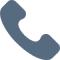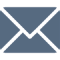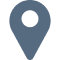# Operations, Measurement, and Thinking (OMT) I

Please note that RMXplore's Math Bytes Courses are not
currently open for registration
.

### Operations, Measurement, and Thinking (OMT) I

Coming Soon!
Course Pricing:
• Online : \$260
• Houston Center : \$320
• Cupertino Center : \$400
Course Logistics:
Duration : to be updated
Number of Classes: 5 weeks course
Occurs : to be updated

### Math Byte Course Topics:

Course Topics are organized as six modules with five granular topics covered under each module. Click through below to see all modules/topics for this course.

#### 01 - Additions and Subtraction• Multiplication of 1-digit numbers
• Division into equal sets
• Repeated subtraction
• Multiplication and division up to 20

#### 02 - Multiplication and Division• Multiplication of 1-digit numbers
• Division into equal sets
• Repeated subtraction
• Multiplication and division up to 20

#### 03 - Measurement• Measuring with a ruler
• Basic unit conversions
• Dealing with money: dollars to cents, etc.
• Telling and writing time at hourly and half-hour marks
• Introduction to length, mass, and volume

#### 04 - Elementary Geometry• Basic shapes: triangle, quadrilateral, pentagon, etc.
• Rectangles and squares
• Circles: what is a radius?
• Recognize shape, size, and color
• Introduction to 3D Objects: cube, block, cylinder, cone

#### 05 - Data Analysis• Organizing data
• Make picture graphs
• Use of symbol/picture to represent one object
• Interpret picture graphs
• Picture graphs with scales

#### 06 - Word Problems